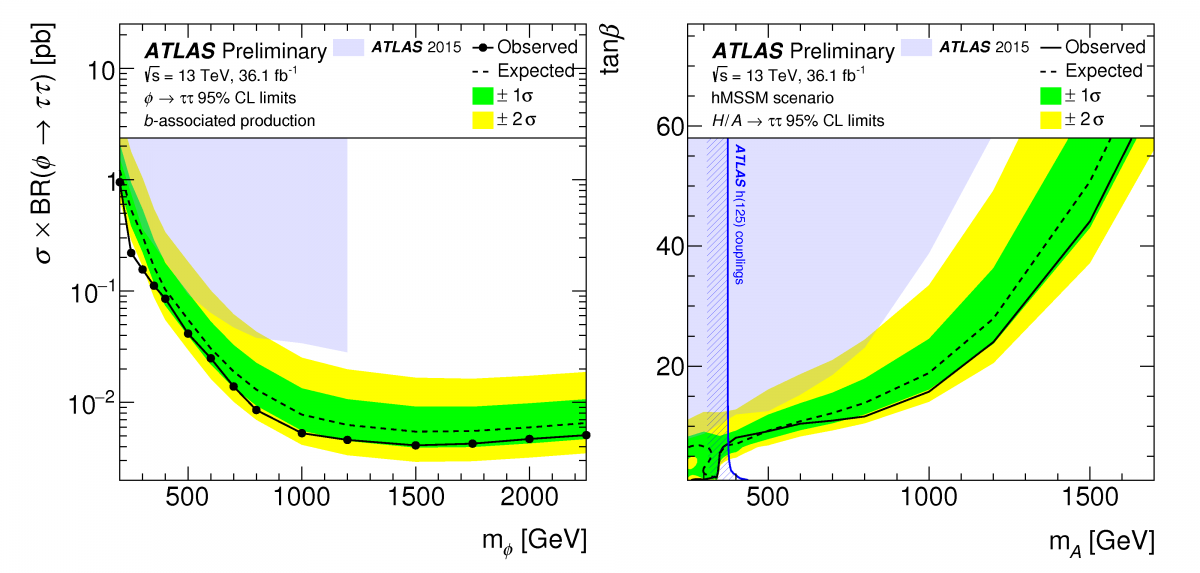Since the discovery of the elusive Higgs boson in 2012, researchers have been looking beyond the Standard Model to answer many outstanding questions. An attractive extension to the Standard Model is Supersymmetry (SUSY), which introduces a plethora of new particles, some of which may be candidates for Dark Matter.Figure 1: Feynman diagram for leading order production of a neutral MSSM Higgs boson in association with b-quarks. (Image: ATLAS Collaboration/CERN)

One of the most popular SUSY models – the Minimal Supersymmetric Standard Model (MSSM) – predicts the existence of five Higgs bosons. In this model, the recently discovered Higgs boson (h) would be considered to be the lightest of the set. Two charged Higgs (H+, H) and two neutral Higgs (A/H) would complete the set, and could exist within a wide range of masses above that of the discovered Higgs boson. The LHC experiments are poised to search for these additional bosons using techniques similar to those used in the initial Higgs searches.

In July 2017, the ATLAS collaboration presented a new result on the search for neutral (A/H) Higgs bosons decaying to two tau leptons. Taus are particularly interesting to the search as there is a stronger coupling between A/H and down-type fermions (e, μ, τ, d, s, b) for certain values of the MSSM parameter-space. This will enhance the probability of decays to tau leptons, as well as the production of A/H in association with b-quarks (Figure 1), providing a larger cross-section. Like with the Standard Model Higgs boson, gluon-fusion production of A/H remains an important production process in the MSSM to varying degrees (depending on the chosen model parameters). Thus, by classifying events by their probability of containing b-flavoured jets, the ATLAS search has been optimised for both b-associated and gluon-fusion production of A/H, respectively.

### One of the most popular SUSY models – the Minimal Supersymmetric Standard Model (MSSM) – predicts the existence of five Higgs bosons.

Since tau leptons decay into lighter particles before reaching the ATLAS detector, a multivariate identification technique is used to identify tau-like clusters. The analysis is further split into events where both taus decay to hadrons, or where one tau decays to leptons and the other into hadrons. Each category has its own challenges, as the background composition depends heavily on the event topology. However, backgrounds in both channels are dominated by quark-initiated jets in the detector that are misidentified as hadronically decaying taus. The probability of such misidentification is measured in control regions enriched in jets.Figure 2 (left): The observed and expected 95% CL upper limits on the production cross section times di-tau branching fraction for a scalar boson produced via b-associated production. Figure 3 (right): The observed and expected 95% CL limits on tanβ as a function of the mass of the A boson in the hMSSM scenario. The area above the black curve has been excluded. The exclusion arising from the Standard Model Higgs boson coupling measurements and the exclusion limit from the ATLAS 2015 H/A→ ττ search are shown. (Images: ATLAS Collaboration/CERN)

The latest ATLAS result shows no sign of the A/H decaying to taus, and places upper limits on the production cross section of A/H via gluon-fusion or b-associated production (Figure 2). These limits can then be cast as exclusion contours in a number of MSSM benchmark scenarios, such as the hMSSM (an MSSM model where the mass of the lightest Higgs boson (h) is fixed at 125 GeV) shown in Figure 3. While large portions of the model phase-space have been excluded, there remains a sizeable area where these additional Higgs bosons could be hiding as the search for SUSY continues.